Caffe
Class List
Here are the classes, structs, unions and interfaces with brief descriptions:
[detail level 1234]
 ▼Ncaffe A layer factory that allows one to register layers. During runtime, registered layers can be called by passing a LayerParameter protobuffer to the CreateLayer function: ►Ndb CAbsValLayer ComputesCAccuracyLayer Computes the classification accuracy for a one-of-many classification task CAdaDeltaSolver CAdaGradSolver CAdamSolver AdamSolver, an algorithm for first-order gradient-based optimization of stochastic objective functions, based on adaptive estimates of lower-order moments. Described in  CArgMaxLayer Compute the index of the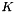max values for each datum across all dimensions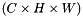CBaseConvolutionLayer Abstract base class that factors out the BLAS code common to ConvolutionLayer and DeconvolutionLayer CBaseDataLayer Provides base for data layers that feed blobs to the Net CBasePrefetchingDataLayer CBatch CBatchNormLayer Normalizes the input to have 0-mean and/or unit (1) variance across the batch CBatchReindexLayer Index into the input blob along its first axis CBiasLayer Computes a sum of two input Blobs, with the shape of the latter Blob "broadcast" to match the shape of the former. Equivalent to tiling the latter Blob, then computing the elementwise sum CBilinearFiller Fills a Blob with coefficients for bilinear interpolation CBlob A wrapper around SyncedMemory holders serving as the basic computational unit through which Layers, Nets, and Solvers interact ►CBlockingQueue CBNLLLayer Computes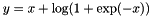if;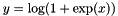otherwise ►CCaffe CConcatLayer Takes at least two Blobs and concatenates them along either the num or channel dimension, outputting the result CConstantFiller Fills a Blob with constant valuesCContrastiveLossLayer Computes the contrastive losswhere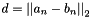. This can be used to train siamese networks CConvolutionLayer Convolves the input image with a bank of learned filters, and (optionally) adds biases CCPUTimer CCropLayer Takes a Blob and crop it, to the shape specified by the second input Blob, across all dimensions after the specified axis CDataLayer CDataTransformer Applies common transformations to the input data, such as scaling, mirroring, substracting the image mean.. CDeconvolutionLayer Convolve the input with a bank of learned filters, and (optionally) add biases, treating filters and convolution parameters in the opposite sense as ConvolutionLayer CDropoutLayer During training only, sets a random portion of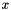to 0, adjusting the rest of the vector magnitude accordingly CDummyDataLayer Provides data to the Net generated by a Filler CEltwiseLayer Compute elementwise operations, such as product and sum, along multiple input Blobs CELULayer Exponential Linear Unit non-linearity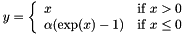CEmbedLayer A layer for learning "embeddings" of one-hot vector input. Equivalent to an InnerProductLayer with one-hot vectors as input, but for efficiency the input is the "hot" index of each column itself CEuclideanLossLayer Computes the Euclidean (L2) lossfor real-valued regression tasks CExpLayer Computes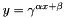, as specified by the scale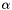, shift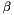, and base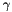CFiller Fills a Blob with constant or randomly-generated data CFilterLayer Takes two+ Blobs, interprets last Blob as a selector and filter remaining Blobs accordingly with selector data (0 means that the corresponding item has to be filtered, non-zero means that corresponding item needs to stay) CFlattenLayer Reshapes the input Blob into flat vectors CGaussianFiller Fills a Blob with Gaussian-distributed valuesCHDF5DataLayer Provides data to the Net from HDF5 files CHDF5OutputLayer Write blobs to disk as HDF5 files CHingeLossLayer Computes the hinge loss for a one-of-many classification task CIm2colLayer A helper for image operations that rearranges image regions into column vectors. Used by ConvolutionLayer to perform convolution by matrix multiplication CImageDataLayer Provides data to the Net from image files CInfogainLossLayer A generalization of MultinomialLogisticLossLayer that takes an "information gain" (infogain) matrix specifying the "value" of all label pairs CInnerProductLayer Also known as a "fully-connected" layer, computes an inner product with a set of learned weights, and (optionally) adds biases CInputLayer Provides data to the Net by assigning tops directly CInternalThread CLayer An interface for the units of computation which can be composed into a Net CLayerRegisterer CLayerRegistry CLogLayer Computes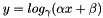, as specified by the scale, shift, and baseCLossLayer An interface for Layers that take two Blobs as input – usually (1) predictions and (2) ground-truth labels – and output a singleton Blob representing the loss CLRNLayer Normalize the input in a local region across or within feature maps CLSTMLayer Processes sequential inputs using a "Long Short-Term Memory" (LSTM)  style recurrent neural network (RNN). Implemented by unrolling the LSTM computation through time CLSTMUnitLayer A helper for LSTMLayer: computes a single timestep of the non-linearity of the LSTM, producing the updated cell and hidden states CMemoryDataLayer Provides data to the Net from memory CMSRAFiller Fills a Blob with values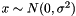where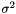is set inversely proportional to number of incoming nodes, outgoing nodes, or their average CMultinomialLogisticLossLayer Computes the multinomial logistic loss for a one-of-many classification task, directly taking a predicted probability distribution as input CMVNLayer Normalizes the input to have 0-mean and/or unit (1) variance CNesterovSolver ►CNet Connects Layers together into a directed acyclic graph (DAG) specified by a NetParameter CNeuronLayer An interface for layers that take one blob as input () and produce one equally-sized blob as output (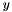), where each element of the output depends only on the corresponding input element CParameterLayer CPoolingLayer Pools the input image by taking the max, average, etc. within regions CPositiveUnitballFiller Fills a Blob with values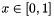such that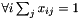CPowerLayer Computes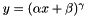, as specified by the scale, shift, and powerCPReLULayer Parameterized Rectified Linear Unit non-linearity. The differences from ReLULayer are 1) negative slopes are learnable though backprop and 2) negative slopes can vary across channels. The number of axes of input blob should be greater than or equal to 2. The 1st axis (0-based) is seen as channels CPythonLayer CRecurrentLayer An abstract class for implementing recurrent behavior inside of an unrolled network. This Layer type cannot be instantiated – instead, you should use one of its implementations which defines the recurrent architecture, such as RNNLayer or LSTMLayer CReductionLayer Compute "reductions" – operations that return a scalar output Blob for an input Blob of arbitrary size, such as the sum, absolute sum, and sum of squares CReLULayer Rectified Linear Unit non-linearity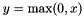. The simple max is fast to compute, and the function does not saturate CReshapeLayer CRMSPropSolver CRNNLayer Processes time-varying inputs using a simple recurrent neural network (RNN). Implemented as a network unrolling the RNN computation in time CScaleLayer Computes the elementwise product of two input Blobs, with the shape of the latter Blob "broadcast" to match the shape of the former. Equivalent to tiling the latter Blob, then computing the elementwise product. Note: for efficiency and convenience, this layer can additionally perform a "broadcast" sum too when bias_term: true is set CSGDSolver Optimizes the parameters of a Net using stochastic gradient descent (SGD) with momentum CSigmoidCrossEntropyLossLayer Computes the cross-entropy (logistic) loss, often used for predicting targets interpreted as probabilities CSigmoidLayer Sigmoid function non-linearity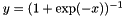, a classic choice in neural networks CSignalHandler CSilenceLayer Ignores bottom blobs while producing no top blobs. (This is useful to suppress outputs during testing.) CSliceLayer Takes a Blob and slices it along either the num or channel dimension, outputting multiple sliced Blob results CSoftmaxLayer Computes the softmax function CSoftmaxWithLossLayer Computes the multinomial logistic loss for a one-of-many classification task, passing real-valued predictions through a softmax to get a probability distribution over classes ►CSolver An interface for classes that perform optimization on Nets CSolverRegisterer CSolverRegistry CSplitLayer Creates a "split" path in the network by copying the bottom Blob into multiple top Blobs to be used by multiple consuming layers CSPPLayer Does spatial pyramid pooling on the input image by taking the max, average, etc. within regions so that the result vector of different sized images are of the same size CSyncedMemory Manages memory allocation and synchronization between the host (CPU) and device (GPU) CTanHLayer TanH hyperbolic tangent non-linearity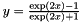, popular in auto-encoders CThresholdLayer Tests whether the input exceeds a threshold: outputs 1 for inputs above threshold; 0 otherwise CTileLayer Copy a Blob along specified dimensions CTimer CUniformFiller Fills a Blob with uniformly distributed values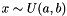CWindowDataLayer Provides data to the Net from windows of images files, specified by a window data file. This layer is DEPRECATED and only kept for archival purposes for use by the original R-CNN CXavierFiller Fills a Blob with values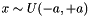where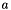is set inversely proportional to number of incoming nodes, outgoing nodes, or their average